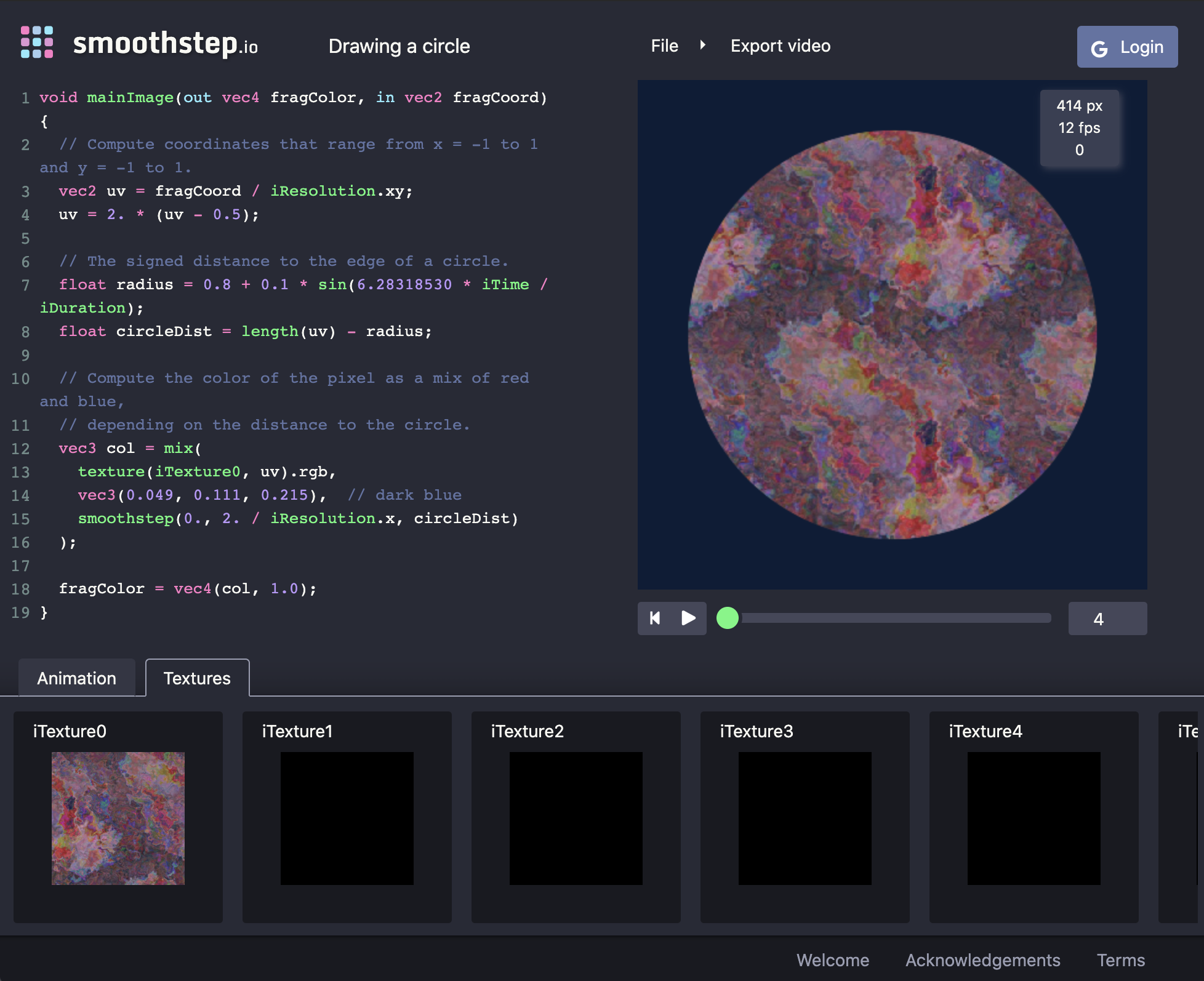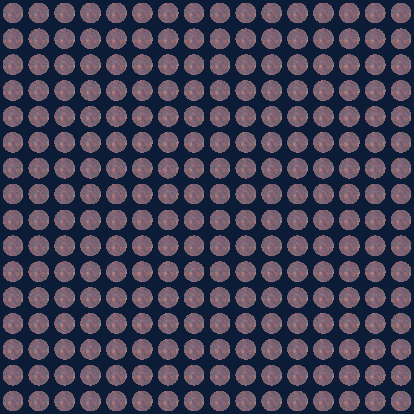# Drawing a Circle

Enter the following code into the editor:

``````void mainImage(out vec4 fragColor, in vec2 fragCoord) {
// Compute coordinates that range from x = -1 to 1 and y = -1 to 1.
vec2 uv = fragCoord / iResolution.xy;
uv = 2. * (uv - 0.5);

// The signed distance to the edge of a circle.
float radius = 0.8 + 0.1 * sin(6.28318530 * iTime / iDuration);
float circleDist = length(uv) - radius;

// Compute the color of the pixel as a mix of red and blue,
// depending on the distance to the circle.
vec3 col = mix(
vec3(0.800, 0.184, 0.277),  // red
vec3(0.049, 0.111, 0.215),  // dark blue
smoothstep(0., 2. / iResolution.x, circleDist)
);

fragColor = vec4(col, 1.0);
}
``````

Click the build button, or press shift+enter to recompile the code. Press play to see a pulsing circle. Read the comments to understand how each pixel is colored depending on whether it is computed to be inside or outside the circle.

## Using textures

Let’s draw the circle using a texture, rather than a flat color. Replace the definition of `col` with:

``````vec3 col = mix(
texture(iTexture0, uv).rgb,  // red
vec3(0.049, 0.111, 0.215),  // dark blue
smoothstep(0., 2. / iResolution.x, circleDist)
);
``````

Recompile, to see a black square. Open the Textures tab, and change `iTexture0` from a black texture to something else.Textures are accessed using the `texture` function, and they are mapped to x = 0 to 1 and y = 0 to 1, repeating at every integer. You can use `textureSize` to get the dimensions of any texture in pixels.

## Manipulating UV Coordinates

A common trick in shader programming is to achieve effects by manipulating the coordinate system itself.

``````void mainImage(out vec4 fragColor, in vec2 fragCoord) {
// Compute coordinates that range from x = 0 to 1 and y = 0 to 1.
vec2 uv = fragCoord / iResolution.xy;

// Manipulate the uv coordinates.
float scale = 2.0;
vec2 offset = vec2(0.5, 0.5);

uv = scale * (uv - offset);

// The signed distance to the edge of a circle.
float radius = 0.8 + 0.1 * sin(6.28318530 * iTime / iDuration);
float circleDist = length(uv) - radius;

// Compute the color of the pixel as a mix of red and blue,
// depending on the distance to the circle.
vec3 col = mix(
texture(iTexture0, uv).rgb,  // red
vec3(0.049, 0.111, 0.215),  // dark blue
smoothstep(0., 2. / iResolution.x, circleDist)
);

fragColor = vec4(col, 1.0);
}
``````

Note that the `uv` coordinates are manipulated with an offset and a scale factor. Play with these values to observe their effects. (Clicking on their values brings up a UI that allows you to dynamically modify them in the code).

We can also cause the image to tile by appling `fract` or `mod` to the `uv` variable. For example, you can draw 16 × 16 circles on the canvas using:

``````// Manipulate the uv coordinates.
float scale = 16.;
uv *= scale;
uv = fract(uv);
uv = 2. * (uv - 0.5);
``````

This is much more efficient than computing `circleDist` for all 256 circles.